# Markov process, stationary

A Markov process which is a stationary stochastic process. There is a stationary Markov process associated with a homogeneous Markov transition function if and only if there is a stationary initial distribution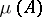corresponding to this function, that is,satisfies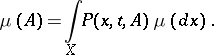If the phase spaceis finite, then a stationary initial distribution always exists, independent of whether the process has discrete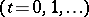or continuous time. For a process in discrete time and for a countable set, a condition for existence of a stationary distribution has been found by A.N. Kolmogorov : It is necessary and sufficient that there is class of communicating states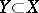such that the mathematical expectation of the time for reachingfromis finite for any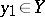. This criterion has been generalized to strong Markov processes with an arbitrary phase space: For the existence of a stationary process it is sufficient that there is a compact set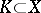such that the expectation of the time of reachingfromis finite for all. There is the following sufficient condition for the existence of a stationary Markov process in terms of Lyapunov stochastic functions (cf. Lyapunov stochastic function): If there is a functionfor whichfor, then there is a stationary Markov process associated with the Markov transition function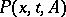. Hereis the infinitesimal generator of the process.
When the stationary initial distributionis unique, the corresponding stationary process is ergodic. In this case the Cesàro mean of the transition probabilities converges weakly to. Under certain additional conditions,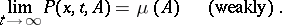A stationary initial distribution satisfies the Fokker–Planck(–Kolmogorov) equation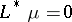, where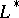is the adjoint operator to the infinitesimal operator of the process. For example,is the adjoint operator to the generating differential operator of the process for diffusion processes. In this casehas a densitywith respect to the Lebesgue measure which satisfies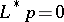. In the one-dimensional case this equation can be solved by quadrature.